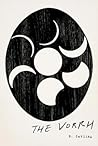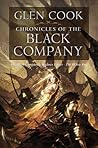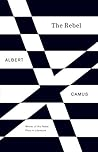Warning: count(): Parameter must be an array or an object that implements Countable in /homepages/2/d410050891/htdocs/wp-includes/post-template.php on line 284

Warning: count(): Parameter must be an array or an object that implements Countable in /homepages/2/d410050891/htdocs/wp-includes/post-template.php on line 284

Warning: count(): Parameter must be an array or an object that implements Countable in /homepages/2/d410050891/htdocs/wp-includes/post-template.php on line 284

Warning: count(): Parameter must be an array or an object that implements Countable in /homepages/2/d410050891/htdocs/wp-includes/post-template.php on line 284

May 29 2012

## Reading Aczel’s Mystery of the Aleph (part 1)

From The Mystery Of The Aleph: Mathematics, The Kabbalah, And The Search For Infinity, by Amir D. Aczel:

“[The ancient Greeks discovered] infinity because of the haunting paradoxes attributed to the philosopher Zeno of Elea (495-435 B.C.). Zeno inferred that motion is impossible under the assumption that space and time can be subdivided infinitely many times.

“[For example,] you can never leave the room in which you are right now. First you walk half the distance to the door, then half the remaining distance, then half of what still remains from where you are to the door, and so on. Even with infinitely many steps – each half the size of the previous one – you can never get past the door! Behind this paradox lies an important concept: even infinitely many steps can sometimes lead to a finite total distance. If each step you take measures half the size of the previous one, then even if you should take infinitely many steps, the total distance traveled measures twice your first distance:

1+1/2+1/4+1/8+1/16+1/32+1/64+…..=2

“Zeno used this paradox to argue that under the assumption of infinite divisibility of space and time, motion can never even start.

***Now. Where this interests me, is that I came up with a similar notion in 8th grade Geometry. We had been talking about infinite numbers, and infinite fractions and decimals. I asked my teacher how we ever arrived at “2”. She told me to shut up, and not ask such questions. I feel vindicated now. :)

Now, let’s turn to our buddy Pythagoras:

“The Pythagorean school at Crotona followed strict code of conduct. The members believed in metempsychosis, the transmigration of souls. Therefore, animals could not be slaughtered for they might shelter the souls of deceased friends. The Pythagoreans were vegetarian and observed additional dietary restrictions.

“The Pythagoreans had a symbol – a five-pointed star enclosed in a pentagon, inside of which was another pentagon, inside it another five-pointed star, and so on to infinity. In this figure, each diagonal is divided by the intersecting line into two unequal parts. The ratio of the larger section to the smaller one is the golden section, the mysterious ratio that appears in nature and in art. The golden section is the infinite limit of the ratio of two consecutive members of the Fibonacci series of the Middle Ages: 1,1,2,3,5,8,13,21,34,55,89,144,233,…. Where each number is the sum of its two previous predecessors. The ratio of each two successive numbers approaches the golden section: 1.618….. This number is irrational. It has an infinite, non-repeating decimal part.

***Also interesting to note, for those of you into connections between Earth and Sirius, the electromagnetic frequency of Earth, in comparison to that of Sirius, is also 1.618…

“The Pythagoreans considered one as the generator of all numbers. This assumption makes it clear that they had some understanding of the idea of infinity, since given any number – no matter how large – they could generate a larger number simply by adding one to it. Two was the first even number, and represented opinion. The Pythagoreans considered even numbers female, and odd numbers male.

*** XX vs. XY?

“Three was the first true odd number, representing harmony. Four, the first square, was seen as a symbol of justice and the squaring of accounts. Five represented marriage: the joining of the first female and male numbers. Six was the number of creation. The number seven held special awe for the Pythagoreans: it was the number of the seven planets or ‘wandering stars.’

“The holiest number of all was ten, tetractys. It represented the number of the universe and the sum of all generators of geometric dimensions: 10=1+2+3+4, where 1 element determines a point (dimension 0), 2 elements determine a line (dimension 1), 3 determine a plane (dimension 2), and 4 determine a tetrahedron (3 dimensions). A great tribute to the Pythagoreans’ intellectual achievements is the fact that they deduced the special place of the number 10 from an abstract mathematical argument, rather than from counting the fingers on two hands.“Ten is a triangular number. Here again we see the strong connection the Pythagoreans saw between geometry and arithmetic. Triangular numbers are numbers whose elements, when drawn, form triangles. Smaller triangular numbers are three and six. The next triangular number after ten is fifteen.

“…The irrationals were a devastating discovery for Pythagoras and his followers because numbers had become the Pythagoreans’ religion. God is number was the cult’s motto. And by numberthey meant whole numbers and their ratios. The existence of the square root of two, a number that could not possibly be expressed as the ratio of two of God’s creations, thus jeopardized the cult’s entire belief system. By the time this shattering discovery was made, the Pythagoreans had become a well-established society dedicated to the study of the power and mystery of numbers.

“Hippasus, one of the members of the Pythagorean order, is believed to have committed the ultimate crime by divulging to the outside world the secret of the existence of irrational numbers.

***Hippasus later died under “mysterious circumstances” (Think “Luka Brozzi sleeps wit’ da fishes…”)

“In a sense, the Pythagoreans’ idea of the divinity of the integers died with Hippasus, to be replaced by the richer concept of the continuum. For it was after the world learned the secret of the irrational numbers that Greek geometry was born. Geometry deals with lines and planes and angles, all of which are continuous. The irrational numbers are the natural inhabitants of the world of the continuum – although rational numbers live there as well – since they constitute the majority of numbers in the continuum. A rational number can be stated in a finite number of terms, while an irrational number, such as Pi (the ratio of the circumference of a circle to its diameter), is intrinsically infinite in its representation: to identify it completely, one would have to specify an infinite number of digits. (With irrational numbers there is no possibility of saying: ‘repeat the decimals 17342 forever,’ since irrational numbers have no patterns that repeat forever.).

“Zeno’s idea of infinity was taken up by two of the greatest mathematicians of antiquity: Eudoxus of Cnidus (408-355 B.C.) and Archimedes of Syracuse (287-212 B.C.)

“In mathematics, Eudoxus used the idea of a limit process. He found areas and volumes of curved surfaces by dividing the area or volume in question into a large number of rectangles or three-dimensional objects and then calculating their sum. Curvature is not easily understood, and to compute it, we need to view a curved surface as the sum of a large number of flat surfaces. Book V or Euclid’s Elements describes this, Eudoxus’s greatest achievement: the method of exhaustion, devised to compute areas and volumes. Eudoxus demonstrated that we do not have to assume the actual existence of infinitely many, infinitely small quantities used in such a computation of the total are or volume of a curved surface. All we have to assume is that there exist quantities ‘as small as we wish’ by the continued division of of any given total magnitude: a brilliant introduction of the concept of potential infinity. Potential infinity enabled mathematicians to develop the concept of a limit, developed in the nineteenth century to establish the theory of calculus on a firm foundation.”

••••